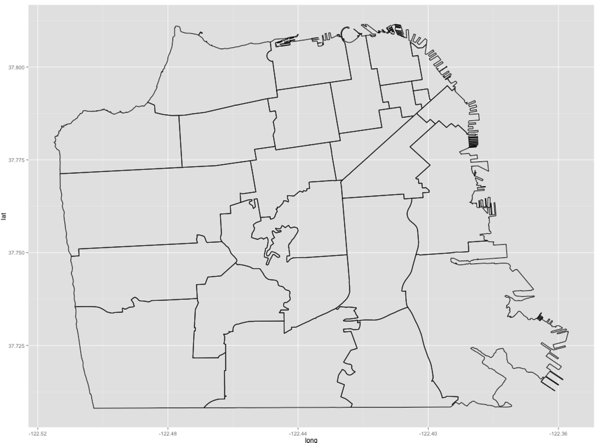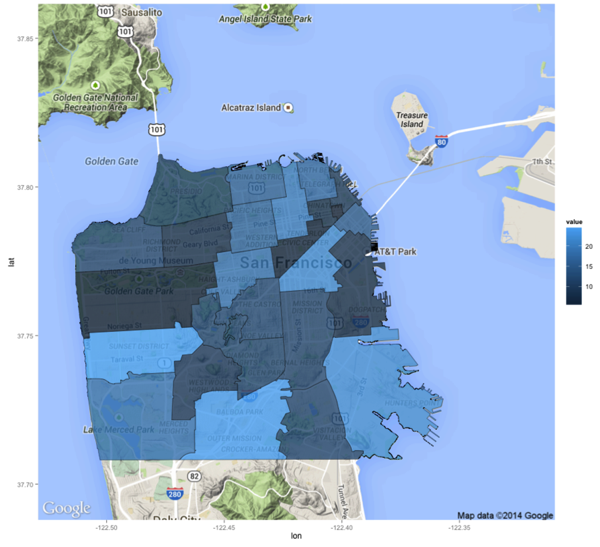# R: ggmap - Overlay shapefile with filled polygon of regions

I’ve been playing around with plotting maps in R over the last week and got to the point where I wanted to have a google map in the background with a filled polygon on a shapefile in the foreground.

The first bit is reasonably simple - we can just import the ggmap library and make a call to get_map:

``````> library(ggmap)
> sfMap = map = get_map(location = 'San Francisco', zoom = 12)``````Next I wanted to show the outlines of the different San Francisco zip codes and came across a blog post by Paul Bidanset on Baltimore neighbourhoods which I was able to adapt.

I downloaded a shapefile of San Francisco’s zip codes from the DataSF website and then loaded it into R using the readOGR and spTransform functions from the rgdal package:

``````> library(rgdal)
> library(ggplot2)
> sfn = readOGR(".","sfzipcodes") %>% spTransform(CRS("+proj=longlat +datum=WGS84"))
> ggplot(data = sfn, aes(x = long, y = lat, group = group)) + geom_path()``````sfn is a spatial type of data frame...

``````> class(sfn)
 "SpatialPolygonsDataFrame"
attr(,"package")
 "sp"``````

...but we need a normal data frame to be able to easily merge other data onto the map and then plot it. We can use ggplot2’s https://groups.google.com/forum/#!topic/ggplot2/PHaeJNq8eNs command to do this:

``````> names(sfn)
 "OBJECTID" "ZIP_CODE" "ID"

> sfn.f = sfn %>% fortify(region = 'ZIP_CODE')

SFNeighbourhoods  = merge(sfn.f, sfn@data, by.x = 'id', by.y = 'ZIP_CODE')``````

I then made up some fake values for each zip code so that we could have different colour shadings for each zip code on the visualisation:

``````> library(dplyr)

> postcodes = SFNeighbourhoods %>% select(id) %>% distinct()

> values = data.frame(id = c(postcodes),
value = c(runif(postcodes %>% count() %>% unlist(),5.0, 25.0)))``````

I then merged those values onto SFNeighbourhoods:

``````> sf = merge(SFNeighbourhoods, values, by.x='id')

Source: local data frame [10 x 10]
Groups: id

id      long      lat order  hole piece   group OBJECTID    ID     value
1  94102 -122.4193 37.77515     1 FALSE     1 94102.1       14 94102  6.184814
2  94103 -122.4039 37.77006   106 FALSE     1 94103.1       12 94103 21.659752
3  94104 -122.4001 37.79030   255 FALSE     1 94104.1       10 94104  5.173199
4  94105 -122.3925 37.79377   293 FALSE     1 94105.1        2 94105 15.723456
5  94107 -122.4012 37.78202   504 FALSE     1 94107.1        1 94107  8.402726
6  94108 -122.4042 37.79169  2232 FALSE     1 94108.1       11 94108  8.632652
7  94109 -122.4139 37.79046  2304 FALSE     1 94109.1        8 94109 20.129402
8  94110 -122.4217 37.73181  2794 FALSE     1 94110.1       16 94110 12.410610
9  94111 -122.4001 37.79369  3067 FALSE     1 94111.1        9 94111 10.185054
10 94112 -122.4278 37.73469  3334 FALSE     1 94112.1       18 94112 24.297588``````

Now we can easily plot those colours onto our shapefile by calling geom_polgon instead of geom_path:

``````> ggplot(sf, aes(long, lat, group = group)) +
geom_polygon(aes(fill = value))``````And finally let’s wire it up to our google map:

``````> ggmap(sfMap) +
geom_polygon(aes(fill = value, x = long, y = lat, group = group),
data = sf,
alpha = 0.8,
color = "black",
size = 0.2)``````I spent way too long with the alpha value set to '0' on this last plot wondering why I wasn’t seeing any shading so don’t make that mistake!